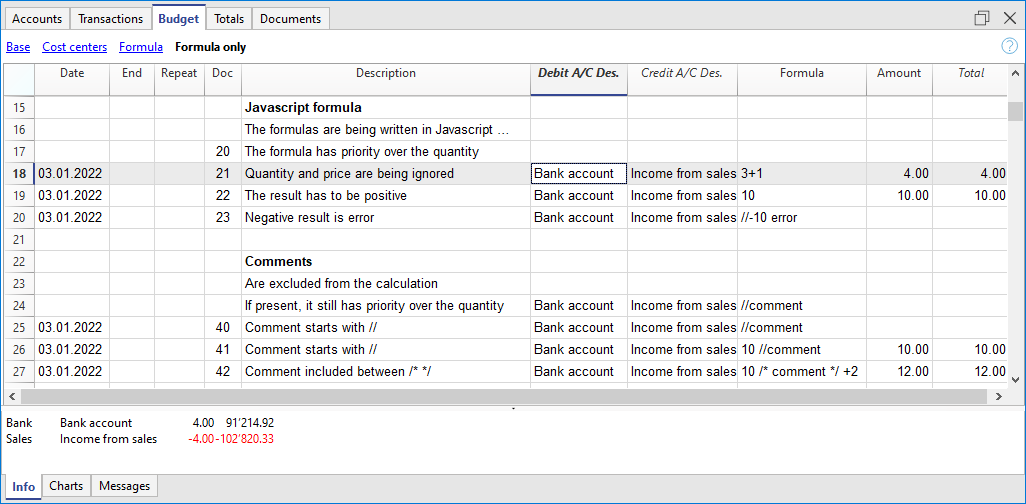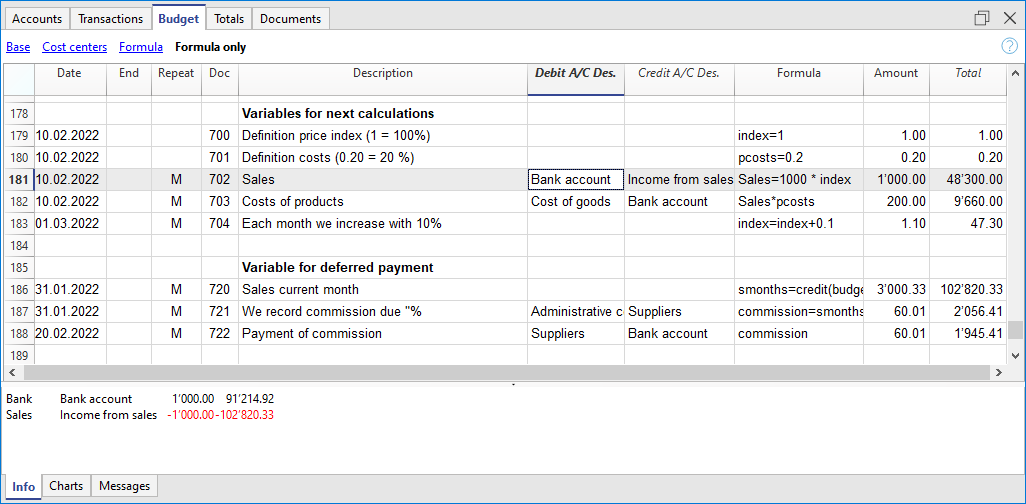# Javascript formulas in the Budget table

The possibility to enter formulas is available in Banana Accounting Plus only with the Advanced plan. Update now!

## Column Formula

The Formula column allows you to enter calculation formulas. The estimate amounts can thus be calculated on the basis of other values of the same budgeting (see Examples of formulas).

• Indicate the cost of goods based as a percentage of sales.
• Increase your sales budget based as a percentage of growth.
• At the end of the year, calculate the depreciation based on the value of the investments made.
• Quarterly, calculate interest on the bank account based on actual usage (days / 365).
• Monthly, calculate interest on the bank account based on actual usage (days / 365).

If you enter a value in the Formula column, the Amount column is calculated by the program based on that formula.

As explained in the documentation of the table Budget, you can use Javascript formulas as well:

• In the Formula Begin column
• In the Description column, for repetitive rows.

## Example files

For examples of the formulas, refer to the following explanations:## Calculation formulas in Javascript

• The formula must be expressed in the Javascript language (not to be confused with the Java language).
• If there is a formula (or any text), the value in the Amount column is set according to the formula result.
• You can use all the functions of the Javascript language, plus the APIs provided by Banana.

## Decimal separator

In JavaScript only the point "." is used as a decimal separator
If you use a different separator, the one used for numbers in the local format, the number is likely to be truncated.

## Amount = result of the last instruction

In Javascript the semicolon ";" is used to separate expressions.
If the Javascript formula contains multiple expressions separated by ";" the value of the Amount column will be the result of the last executed expression.

• 10*3 //30 will be returned
• If there is a sequence of several operations separated by a semicolon ";", the last operation will be resumed.
10*3;7; //7 will be returned
• If there is a return, the value is resumed after the return.
return 10; // 10 will be returned.

## Variables

Javascript variables are the most powerful elements of programming, as they allow you to access the computer memory with a name, save and access those values.
Variables do not exist in Excel formulas, but are similar to the name of the cells, with the difference that the name can be freely assigned.The variable is created by indicating a name and indicating with the equal sign (=).

You can define and use variables directly within the rows. By entering the variable name in the formula, the saved value is taken over.

The price variable is created here and the  value 10 is assigned.

`var price = 10;`

or simply

`price = 10;`

below we create a total variable to which the price value multiplied by 5 is attributed.

total = price * 5;

The value of the variable can be reassigned.

price = 20;

### Objects

Javascipt objects are variables that allow you to save multiple values, each indicated with a property.
The prices object is created below, indicating the curly brackets. To access and save the values, instead use the square brackets or indicate in the name of the property after the name of the object.

```prices = {}
prices['car'] = 10;
prices.car = 10;
prices['computer'] = 20;
prices.computer = 20;
```

### Array

Javascript Arrays are created using square brackets and also to access them.
The first element of the array has index 0.

```costs = [1,2,3];
costs=3;
result = prices['car'] - costs;```

## Repetition and variables

For more information on the calculation sequence, see Planning Logic.

• All rows (including those created for repetitions) are sorted by date. If there are rows with the same date, the order is that of insertion in the table.
• After the sorting, the rows (including the formulas) are recalculated.
• In the formulas, you therefore only have access to the budget data up to that date.
• A variable must be defined in a row that has the date preceding the row where it is used.
• If the budget entry has a repetition the variable will be reassigned each time.
sum = 10;
• If you want to calculate the grand total instead.
• In an initial line create the variable with value zero.
sum = 0;
• In the line that repeats in the sum also include the previous value.
sum = sum + 10;
or use the "+="
sum += 10

## Automatic variables

• budgetCurrent
It is a table that contains the budget rows after the repetitions creation.
These are used to record values in conjunction with the JRepeatNumber.
• DEBUG  is a variable that can be "true" or "false".
If "true", in the messages, all the results of the formulas are being displayed.
• row
Is a Javascript object that refers to the current row.
• The values of the cells can be retrieved with the value function ("columnNameXml").
row.value("date") returns to the date of the transaction.
• row.value ("JRepeatNumber") returns the progressive of the repetition.
The first repetition is 0.
• _totalPrice
This is the value of the Quantity column multiplied by the UnitPrice column.
Equivalent to the formula "row.value('Quantity')*row.value('UnitPrice')"

## Budget Functions

In addition to the budget API defined in the accounting class API, there are specific functions.

##### BudgetGetPeriod(tDate, period)

This function is used in combination with the use of repetition.
When repetitions are indicated, it is advisable to refer to a calculation period and not to a precise date.

• Parameter tDate.
The date to which the calculation of the period refers. Usually the date of the recording line.
• Period parameter.
Abbreviations
• For the current month
• "MC" current month
• "QC" current trimester
• "YC" current year.
• For previous month
• "MP" previous month
• "QP" previous quarter
• "YP" previous year.
• In the Experimental version (starting from version 9.1) can also be used
• "DC" current day
• "DP" previous day
• "WC" current week
• "WP" previous week
• "BC" current bi-monthly (2 months)
• "BP" previous bi-monthly
• "SC" current half-year
• "SP" previous shalf-year
•  Returned values.
An object composed of two dates
• startDate
• endDate
```// example
t = BudgetGetPeriod ('2015-01-01', 'MP') returns
t.startDate // 2014-12-01
t.endDate // 2014-12-31```

## Specific budget functions

The following are similar to those available with Banana.document, but can be used without indicating the object Banana.document.

To be taken into account:

• Instead of the startDate parameter, you can use one of the abbreviations "MC", "MP", explained in the budgetGetPeriod.
• If you use an abbreviation, the function calculates the start and end date of the period, based on the date of the current registration.
• It makes sense to use the end date only if it is earlier than the row date.
If it is equal or higher, it has no effect because the values after the current row are not yet available, because they have not been processed.
• If the registration row date is April 15th:
• budgetBalance("1000","MP") the balance of the 1000 account returns at the end of March.
• budgetBalance("1000","MC") returns the balance at the current time is the same as budgetBalance("1000").
• budgetTotal("1000","QP") returns total of the movement for the previous quarter.
• budgetTotal("1000","QC") returns the total of the movement for the previous quarter, up to the current date.
##### budgetBalance(account, startDate, endDate, extraParam)

The balance up to the current row.

`budgetBalance('1000', 'MP'); //returns the balance of 1000 at the end of the previous month`
##### budgetOpening(account, startDate, endDate, extraParam)

The balance at the beginning of the period.

##### budgetTotal(account, startDate, endDate, extraParam)

The difference between the debit and the credit movement of the period.

`budgetTotal('1000', 'MC'); //returns the total movement of the 1000 account for the current month`
##### budgetInterest( account, interest, startDate, endDate, extraParam)

Calculates the interest on an account, for the period indicated (at the maximum the current date)
If you use it to calculate interest on an account at the end-of-period, the row where the formula is shown should always be the last one for this date.

• Account parameter
This is the account number on whose movements interest will be calculated
• Interest parameter,
Indicates the interest rate in percent.
• Positive (2.5, 4, 10) calculates the interest on the account's debit movement
• Negative (-2.5, -4, -10) calculates the interest on the account's credit movement.
##### credit(amount)
• If the amount parameter is negative returns the amount as a positive
credit(-100) // returns 100

• If the amount parameter is positive, returns 0 (zero)
credit(100) // returns 0

This function is useful in conjunction with the other budgetBalance functions to work only on the balances you need.
If you want to calculate the percentage on sales, using this function is easier.
credit(budgetTotal('1000')) // enter the value only if it is a negative.

##### debit(amount)
• If the amount parameter is positive, returns the amount
debit(100) // returns 100

• If the amount parameter is negative, returns 0 (zero)
debit(-100) // returns 0

Useful if you have to make calculations using only the debit amount and avoid using the credit amount

##### include

Includes and executes a Javascript file, with the possibility to create its own functions and variables that can be recalled in the script.

• Include "file:test.js"
Executes the contents of the indicated file. The name refers to the file on which one is working.
• Include "documents:test.js"
Executes the contents of the text document contained in the documents table.
This has to be a file of the "text/javascript" type.

## Functions for multi-currency accounting

They can also be used for accounting without multi-currency, in this case the account is always in basic currency.

##### budgetBalanceCurrency(account, startDate, endDate, extraParam)

The balance in the account currency up to the current line.

##### budgetExchangeDifference (account, [date, exchangeRate])

This formula recalls the Banana.document.budgetExchangeDifference function.

##### budgetOpeningCurrency(account, startDate, endDate, extraParam)

The balance in the account currency at the beginning of the period.

##### budgetTotalCurrency(account, startDate, endDate, extraParam)

Starting with version 9.01, the following functions are also included : budgetCreditCurrency, budgetDebitCurrency.

### User defined functions

The user can define personal function with the JavaScript language, and recall them in the formulas.
It is possible to define functions:

• Directly in a formula
• In a JavaScript coded attachment; this attachment must have as row id the "_budget.js" name
• In a text of the Documents table, that needs to be included with the Include command

Note:
In the user functions it is currently not possible to use functions that access current accounting data (currentBalance, ...).

```function Taxcalculation(profit)
{
var percentage = 10;
if (profit > 50000)
percentage = 10;
else if (profit > 100000)
percentage =20;
return profit * percentage / 100;
}
```

#### Help us improve the documentation

We welcome feedback on how to improve this page.

Tell us what theme needs a better explanation or how to clarify a topic.